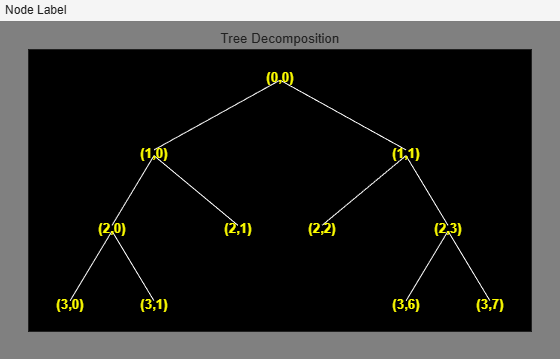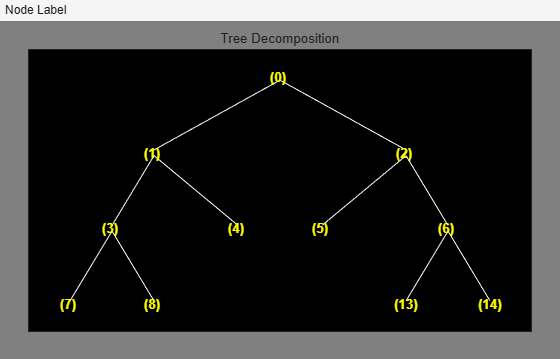# leaves

Determine terminal nodes

## Syntax

``N = leaves(T)``
``[N,K] = leaves(T,"sort")``
``N = leaves(T,"dp")``
``[N,K] = leaves(T,"sortdp")``

## Description

````N = leaves(T)` returns the indices of the terminal nodes of the tree `T` in the column vector `N`. The nodes are ordered from left to right as in the tree.```
````[N,K] = leaves(T,"sort")` returns the sorted indices of the terminal nodes of the tree `T`. `M = N(K)` are the indices reordered as in the tree, from left to right.```[N,K] = leaves(T,"sort")``` and ```[N,K] = leaves(T,"s")``` are equivalent.```
````N = leaves(T,"dp")` returns the depths and positions of the terminal nodes. `N(i,1)` is the depth and `N(i,2)` is the position of the ith terminal node.```

example

````[N,K] = leaves(T,"sortdp")` returns the depths and positions of the sorted terminal nodes.```[N,K] = leaves(T,"sortdp")``` and ```[N,K] = leaves(T,"sdp")``` are equivalent.```

## Examples

collapse all

Create a binary tree of depth 3.

```ord = 2; t = ntree(ord,3);```

Merge the nodes at indices 4 and 5. Plot the result.

```t = nodejoin(t,5); t = nodejoin(t,4); fig = plot(t);```Programmatically, in Node Label, change the selected item from `Depth_Position` to `Index`.

`plot(t,"setNodeLabel",fig,"Index")`List the indices of the terminal nodes.

`tnodes_ind = leaves(t) %#ok<NASGU>`
```tnodes_ind = 6×1 7 8 4 5 13 14 ```

List the terminal nodes, this time sorted on the index.

`[tnodes_ind,Ind] = leaves(t,"sort") %#ok<ASGLU>`
```tnodes_ind = 6×1 4 5 7 8 13 14 ```
```Ind = 6×1 3 4 1 2 5 6 ```

List the depths and positions of the terminal nodes.

`tnodes_depo = leaves(t,"dp") %#ok<NASGU>`
```tnodes_depo = 6×2 3 0 3 1 2 1 2 2 3 6 3 7 ```

List the depths and positions again, this time, sorted on depth and position.

`[tnodes_depo,Ind] = leaves(t,"sortdp")`
```tnodes_depo = 6×2 2 1 2 2 3 0 3 1 3 6 3 7 ```
```Ind = 6×1 3 4 1 2 5 6 ```

## Input Arguments

collapse all

Tree, specified as a `ntree`, `dtree`, or `wptree` object.

## Output Arguments

collapse all

Terminal node description, returned as a column vector or matrix. The nodes are numbered from left to right as in the tree `T`. The root index is 0.

• If ```N = leaves(T)```, `N` is a P-by-1 column vector, where P is the number of terminal nodes in the tree. `N(i)` is the index of the ith node.

• If ```N = leaves(T,"dp")```, `N` is a P-by-2 matrix. `N(i,1)` is the depth and `N(i,2)` is the position of the ith node.

Indices, returned as a vector. The indices `K` are such that ```M = N(K)``` are the terminal indices reordered from left to right.

## Version History

Introduced before R2006a